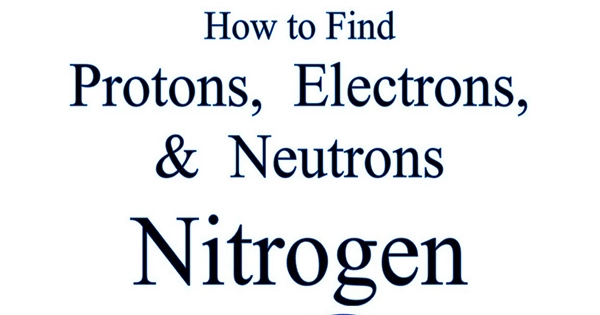Physics

# How Many Protons, Neutrons, and Electrons Make up an Atom of Nitrogen-15?Hint To maintain the atom’s electrical neutrality, the number of protons must always equal its atomic number or Z, and the number of electrons must always equal the number of protons. We may determine the number of neutrons by using the formula Z+N=A. You can now respond to the supplied statement appropriately and with ease.

An isotope of the nitrogen atom called nitrogen-15 is created when the nitrogen atom obtains a neutron in its nucleus. The substances known as isotopes are those with the same atomic number but a different mass number. Thus, nitrogen has an atomic number of 7 and a mass number of nitrogen-15.

The atomic number, or Z, is equal to the number of protons in an atom. Additionally, since we know that nitrogen has an atomic number of 7, this means that nitrogen has seven protons.

Z+N=A
Here, Z is the atomic number.
N represents the number of neutrons
A is the atomic mass of the atom.
As we know that;
Z=7
And A=15 (given)
Then;
7+N=15N=15−7=8
So, the number of neutrons in the nitrogen atom is eight.

Thus, one atom of nitrogen-15 is made up of seven protons, eight neutrons, and seven electrons.

The positively charged protons, negatively charged electrons, and neutral neutrons make up an atom. Protons are positively charged, electrons are negatively charged, and neutrons are neutral particles.